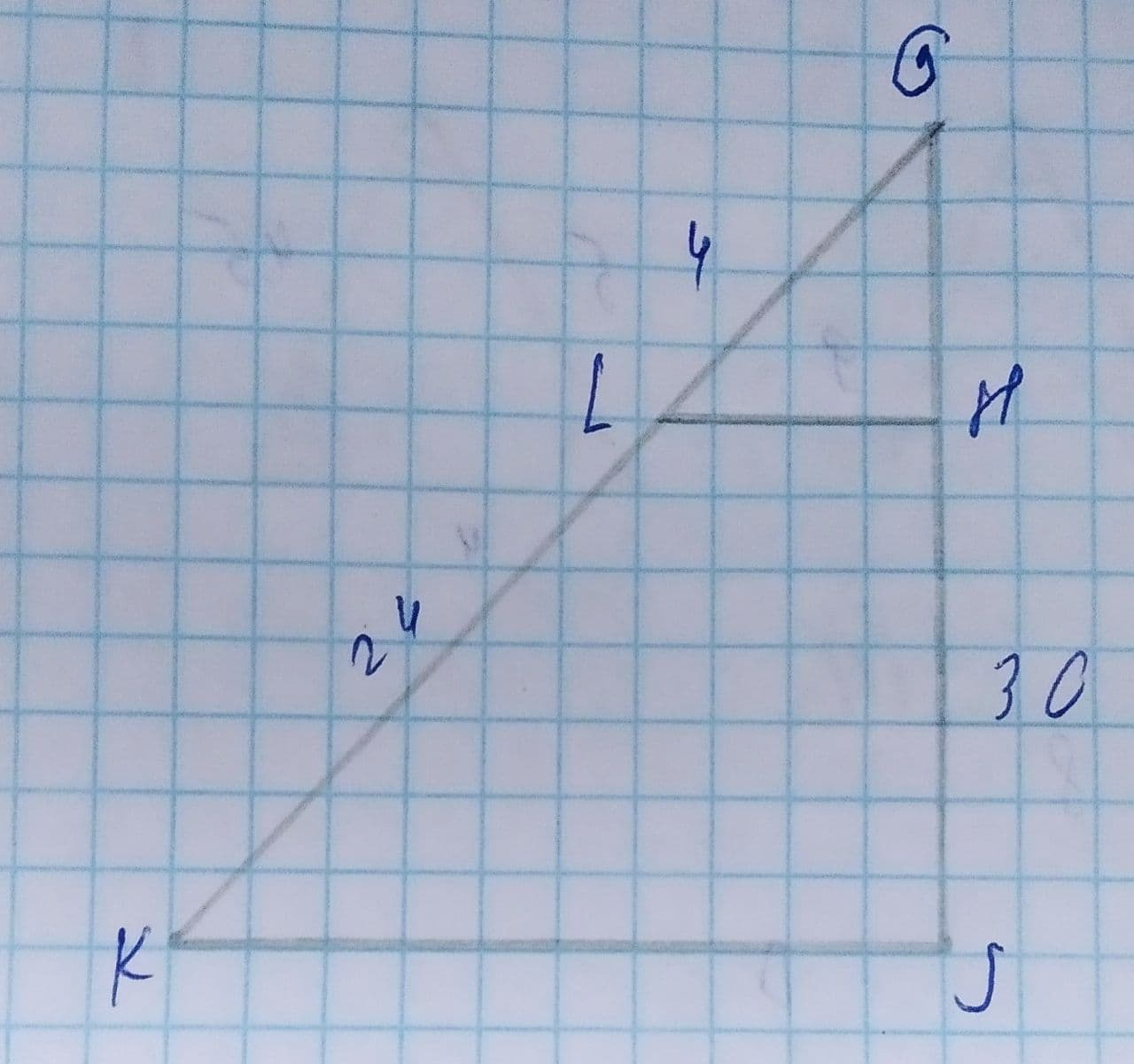Question# In the diagram of \triangle GKJ below, LH\parallel KJ, GL=4, LK=24, and HJ=30. What is the lenght of GJ?

Similarity
ANSWEREDIn the diagram of $$\displaystyle\triangle{G}{K}{J}$$ below, $$\displaystyle{L}{H}\parallel{K}{J}$$, GL=4, LK=24, and HJ=30. What is the lenght of GJ?2021-08-09
Step 1
Here, the objective is to find the length of the side using the similarity of the triangle.
Step 2
In the figure, check the similarity of the triangles $$\displaystyle\triangle{G}{L}{H}\ {\quad\text{and}\quad}\ \triangle{G}{K}{J}$$.
Here, $$\displaystyle{L}{H}{p}{a}{r}{a}{l}\le{l}{K}{J}$$, therefore,
$$\displaystyle\angle{G}{L}{H}=\angle{G}{K}{J}$$
$$\displaystyle\angle{G}{H}{L}=\angle{G}{J}{K}$$
$$\displaystyle\angle{L}{G}{H}=\angle{K}{G}{J}$$
Therefore, both the triangles $$\displaystyle\triangle{G}{L}{H}\approx\triangle{G}{K}{J}$$
So, the ratio of sides of the triangles will be equal.
Step 3
Now, use the ratio of the sides as,
$$\displaystyle{\frac{{{G}{L}}}{{{L}{K}}}}={\frac{{{G}{H}}}{{{H}{J}}}}$$
$$\displaystyle{\frac{{{4}}}{{{24}}}}={\frac{{{G}{H}}}{{{30}}}}$$
GH=5
The length of the side GJ is,
GJ=GH+HJ
=5+30
=35
Therefore, the length of side GJ=35